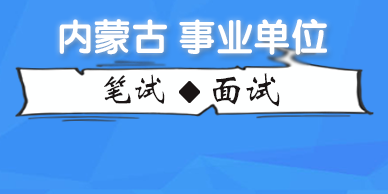内蒙古华图

+ 关注
QQ群

# 2020内蒙古事业单位考试备考:和差数列

2020-02-14 00:56:41 内蒙古事业单位考试网 http://nmg.huatu.com/sydw/ 文章来源：华图教育

【导读】华图内蒙古事业单位考试网同步华图教育发布：2020内蒙古事业单位考试备考:和差数列,详细信息请阅读下文!考试交流，政策解读，备考资料下载请加【2019内蒙古事业单位考试交流QQ群】 ，更多资讯请关注内蒙古华图微信公众号(nmghuatu),内蒙古事业单位考试培训咨询电话：0471-3248222.

2020内蒙古事业单位考试备考:和差数列

在解答公务员录用考试、事业单位公开招聘考试、大学生村官考试等公职考试的行政职业能力测验考试中数字推理题时，考生应明确一种观点，即做数字推理题的基本思路是“尝试错误”。很多数字推理题都不能一眼就看出规律，找到答案，而是要经过两三次的尝试，逐步排除错误的假设，最后才能找到正确的规律。考生能熟练运用一些基本题型的解题规律才能快速、准确地解答数字推理题。在本文中华图教育总结、归纳了和差数列及其变式的基本题型的解题技巧与规律，并通过实例来说明其应用。

(一)和差数列

和差数列的主要特点为第三项是由前两项产生的，故只要第三项与前两项存在某种联系且变化幅度不是很大，就可以考虑和差数列的规律。

【例1】1，2，3，5，8，13，()。

A.14B.15C.20D.21

【解答】 本题正确答案为D。这是一个和数列。前两项之和等于第三项，即1+2=3，2+3=5，3+5=8，5+8=13，故空缺项为8+13=21，选D。

【例2】17，10，()，3，4，-1。

A.7B.6C.8D.5

【解答】 本题正确答案为A。这是一个差数列。前两项之差等于第三项。即17-10=7，7-3=4，3-4=-1。所以正确答案为A项。

(二)和差数列的变式

如果某个数列的前两项相加或相减后再经过某种变化得到第三项，则这个数列为和差数列的变式。这种变化可能是加、减、乘、除某一个常数，或者与项数之间具有某种关系等情况。

【例3】22 ， 35 ， 56 ， 90 ， () ， 234。

A.162B.156C.148D.145

【解答】本题正确答案为D。这是一个和差数列的变式。注意观察前两项与第三项的关系：(22+35)-1=56， (35+56)-1=90，(56+90)-1=145， (145+90)-1=234。由此可知，D项是正确答案。

【例4】4，8，6，7，()，27/4。

A.13B.13/2C.17D.214

【解答】 本题正确答案为B。这是一个和差数列的变式。观察前两项与第三项的关系：(4+8)÷2=6，(8+6)÷2=7，(6+7)÷2=13/2，(7+13/2)÷2=27/4。由此可知，正确答案是B项。

【例5】4，5，11，14，22，()。

A.24B.26C.27D.36

【解答】 本题正确答案为C。这是一个和差数列的变式。每相邻两项之和构成一个平方数列：4+5=9=3^2，5+11=16=4^2，11+14=25=5^2，14+22=36=6^2。则空缺处应为7^2-22=49-22=27。

(三)三项和数列及其变式

三项和数列是数字推理部分出现的一种新题型。它的基本特点是“三项之和为第四项”。三项和数列的变式是指三项之和经过变化之后得到第四项，这种变化可能是加、减、乘、除某一常数，或者每三项之和与项数之间具有某种关系。

【例6】0，1，1，2，4，7，13，()。

A.22B.23C.24D.25

【解答】 本题正确答案为C。这是一个三项和数列，即前三项之和为第四项。0+1+1=2，1+1+2=4，1+2+4=7，2+4+7=13，故空缺处应为4+7+13=24，所以，正确答案为C项。

【例7】2，3，4，9，12，15，22，()。

A.27B.31C.36D.42

【解答】 本题正确答案为A。这是一个三项和数列的变式。它的规律是每相邻的三项之和构成一个平方数列：2+3+4=9=3^2，3+4+9=16=4^2，4+9+12=25=5^2，9+12+15=36=6^2，12+15+22=49=7^2。故空缺处应为8^2=64-(15+22)=27，所以正确答案为A项。——相关阅读——（编辑：bpf）10万+

30w+

1000+• 内蒙古总部
• 呼和浩特市
• 包头市
• 鄂尔多斯市
• 乌兰察布市
• 巴彦淖尔市
• 乌海市
• 阿拉善盟
• 锡林郭勒盟
• 赤峰市
• 通辽市
• 兴安盟
• 呼伦贝尔盟